## Excel BINOM.DIST function

The BINOM.DIST function returns the individual term binominal distribution probability, which can be used to calculate the probability of a specified number of successes out of a given number of trials.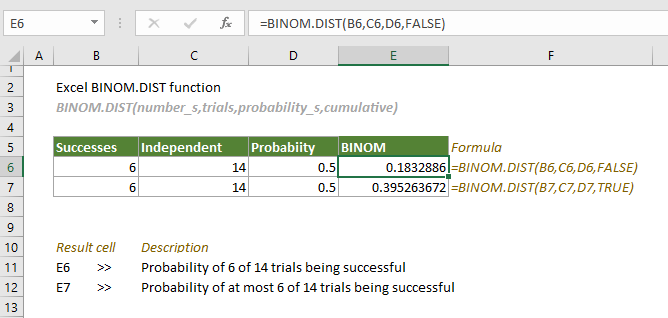#### Syntax

BINOM.DIST(number_s, trials, probability_s, cumulative)

#### Arguments

• Number_s (required): The number of successes in trials;
• Trials (required): The number of independent trials;
• Probability_s (required): The probability of success per trial;
• Cumulative (required): A logical value used to determine the form of the function. It can be:
-- TRUE: use the cumulative distribution function;
-- FLASE: use the probability mass function.

#### Remarks

1. If the arguments “number_s” and “trials” are not integers, they will be truncated;
2. The #NUM! error value occurs when any of the following conditions is met:
-- “Number_s” < 0;
-- “Number_s” > “trials”;
-- “Probability_s” < 0, or “Probability_s” > 1;
3. The #VALUE! error value occurs if any of the supplied “number_s”, “trials” or “probability_s” are non-numeric;
4. Supposing x = number_s, n = trials, and p = probability_s, the equations for the cumulative distribution function and the probability mass function are as follows:
-- The equation for the cumulative distribution function: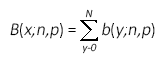-- The equation for the probability mass function: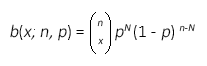where: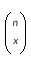is COMBIN(n,x).

#### Return value

It returns a numeric value.

#### Example

As shown in the table below, there are 6 successes in 14 trials, and the probability of success in each trial is 0.5. To calculate the probability of at most 6 successes out of 14 trials and the probability of 6 successes out of 14 trials separately, you can apply the BINOM.DIST function as follows.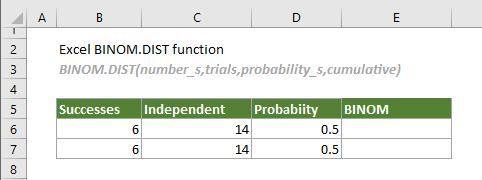1. Calculate the probability of exactly 6 successes out of 14 trials.

Select a cell (E6 in this case), copy or enter the formula below and press Enter to get the result.

=BINOM.DIST(B6,C6,D6,FALSE)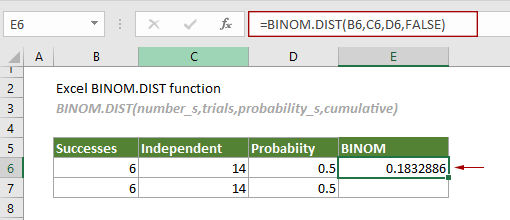2. Calculate the probability of at most 6 successes out of 14 trials.

Select a cell (E7 in this case), copy or enter the formula below and press Enter to get the result.

=BINOM.DIST(B7,C7,D7,TRUE)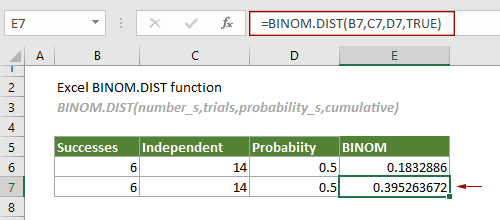#### Related Functions

Excel BINOMDIST function
The BINOMDIST function returns the individual term binominal distribution probability.

### The Best Office Productivity Tools

#### Kutools for Excel - Helps You To Stand Out From Crowd

 Popular Features: Find, Highlight or Identify Duplicates  |  Delete Blank Rows  |  Combine Columns or Cells without Losing Data  |  Round without Formula ... Super VLookup: Multiple Criteria  |  Multiple Value  |  Across Multi-Sheets  |  Fuzzy Lookup... Adv. Drop-down List: Easy Drop Down List  |  Dependent Drop Down List  |  Multi-select Drop Down List... Column Manager: Add a Specific Number of Columns  |  Move Columns  |  Toggle Visibility Status of Hidden Columns  |  Compare Columns to Select Same & Different Cells ... Featured Features: Grid Focus  |  Design View  |  Big Formula Bar  |  Workbook & Sheet Manager | Resource Library (Auto Text)  |  Date Picker  |  Combine Worksheets  |  Encrypt/Decrypt Cells  |  Send Emails by List  |  Super Filter  |  Special Filter (filter bold/italic/strikethrough...) ... Top 15 Toolsets:  12 Text Tools (Add Text, Remove Characters ...)  |  50+ Chart Types (Gantt Chart ...)  |  40+ Practical Formulas (Calculate age based on birthday ...)  |  19 Insertion Tools (Insert QR Code, Insert Picture from Path ...)  |  12 Conversion Tools (Numbers to Words, Currency Conversion ...)  |  7 Merge & Split Tools (Advanced Combine Rows, Split Excel Cells ...)  |  ... and more

Kutools for Excel Boasts Over 300 Features, Ensuring That What You Need is Just A Click Away...#### Office Tab - Enable Tabbed Reading and Editing in Microsoft Office (include Excel)

• One second to switch between dozens of open documents!
• Reduce hundreds of mouse clicks for you every day, say goodbye to mouse hand.
• Increases your productivity by 50% when viewing and editing multiple documents.
• Brings Efficient Tabs to Office (include Excel), Just Like Chrome, Edge and Firefox.